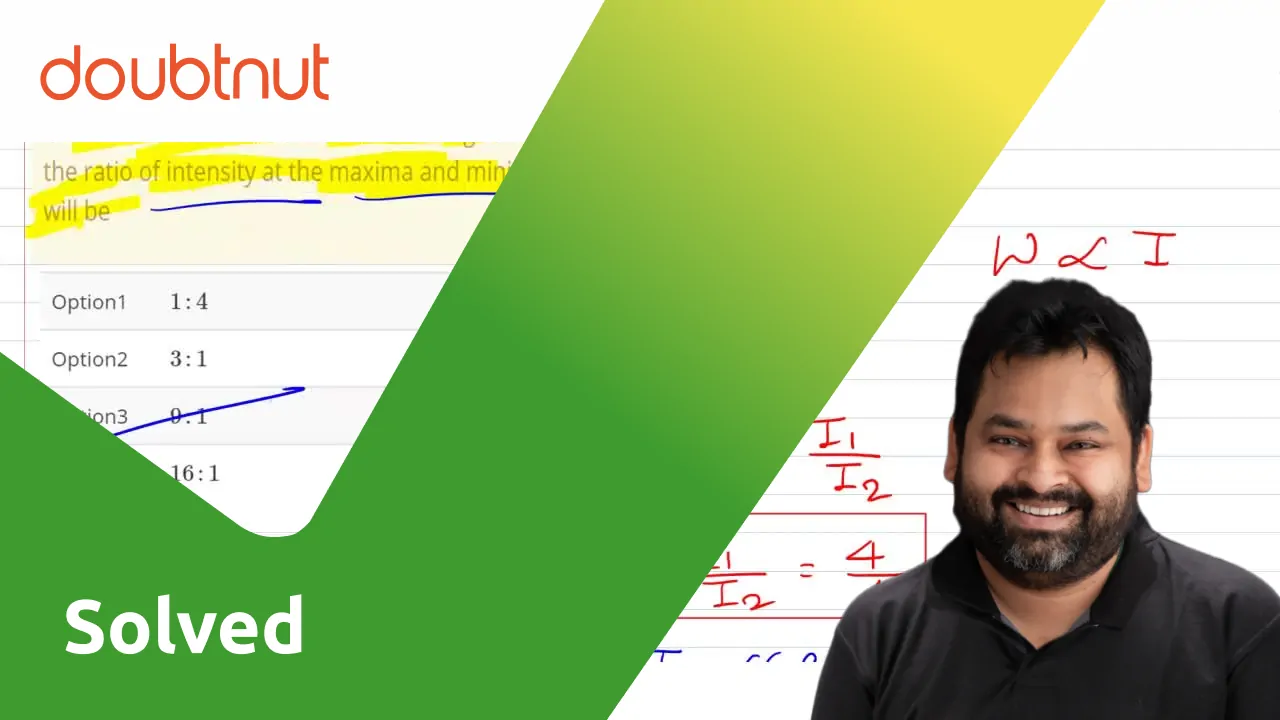Doubtnut App - Get 50 Lakh+ video solutions better than GoogleInstall Now

# If the width ratio of the two slits in Young's double slit experiment is 4:1, then the ratio of intensity at the maxima and minima in the interference patternn will be

A

1:4

B

3:1

C

9:1

D

16:1

Text Solution
Verified by Experts

## W1W2=I1I2=41=a21a22 ∴a1=2a2 ImaxImin=(a1+a2)2(a1−a2)2=(3a2a2)2=91